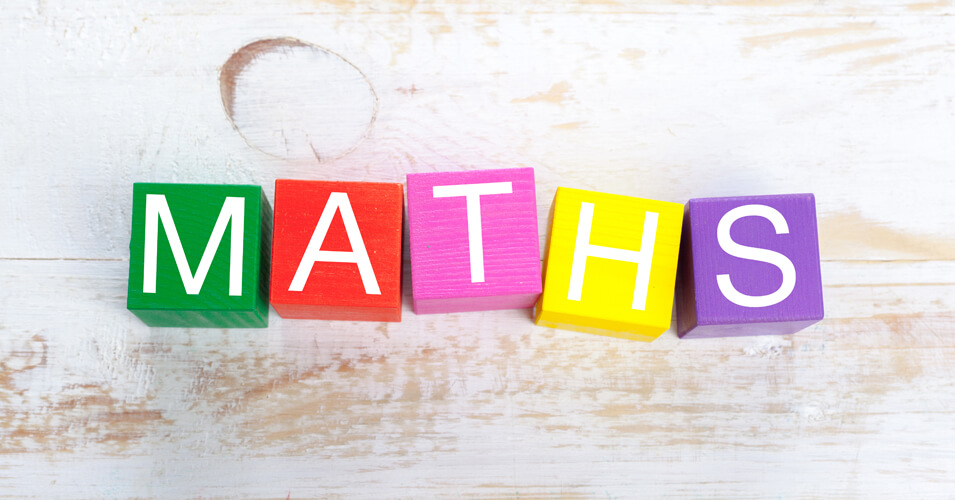• Save

# Maths Questions Series #5

5 months ago 30.9K Views## Very important Maths questions for SSC CGL

Q. The product of 2 numbers is 1575 and their quotient is 9/7. Then the sum of the numbers is –
a. 74
b. 78
c. 80
d. 90

Q. The value of (81)3.6 * (9)2.7/ (81)4.2 * (3) is __
a. 3
b. 6
c. 9
d. 8.2

Q. ?6+?6+?6+… is equal to –

a. 2
b. 5
c. 4
d. 3

Q. The sum of the squares of two natural consecutive odd numbers is 394. The sum of the numbers is –

a. 24
b. 32
c. 40
d. 28

Q. When (6767 +67) is divided by 68, the remainder is-

a. 1
b. 63
c. 66
d. 67

Q. In a division sum, the divisor is 4 times the quotient and twice the remainder. If a and b are respectively the divisor and the dividend then-

a. 4b-a2 /a =3
b. 4b-2a /a2 =2
c. (a+1)2 = 4b
d. A(a+2)/b = 4

Q. If 738 A6A is divisible by 11, then the value of A is-

a. 6
b. 3
c. 9
d. 1

## About author

### Vikram Singh

Providing knowledgable questions of Reasoning and Aptitude for the competitive exams.

## Report Error: Maths Questions Series #5

Please Enter Message
Error Reported Successfully